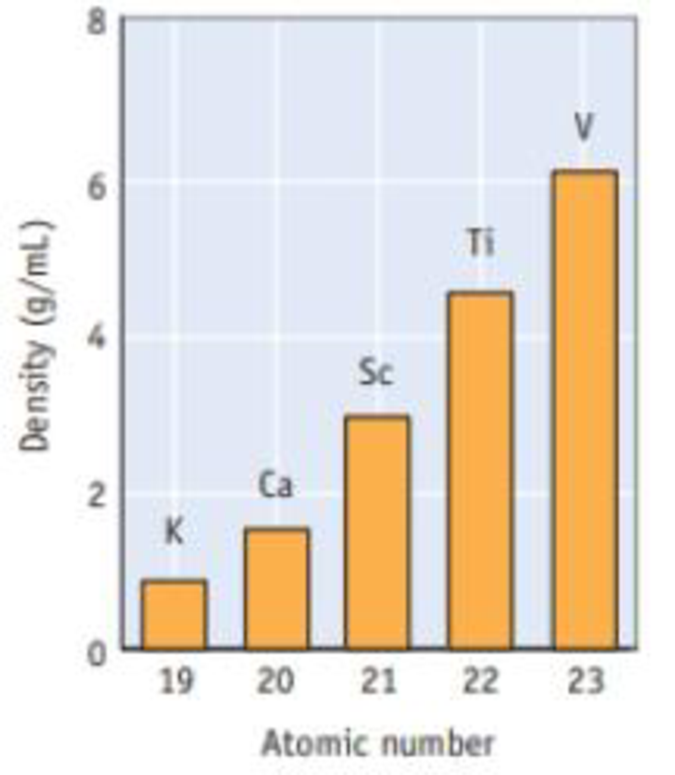Chapter 7, Problem 81SCQ

Chapter
Section
Textbook Problem

Using your knowledge of the trends in element sizes on going across the periodic table, explain briefly why the density of the elements increases from K through V.Interpretation Introduction

Interpretation:

The density of the elements increases from K through V; the reason has to be explained.

Concept Introduction:

Atomic radius: The concept explain chemical element is a measured of the size of its atoms, in other words to measure the distance from the center of the nucleus to the boundary of the surrounding cloud of number of electrons.

Increase and decrease electro negativity: The less vacancy electrons an atoms has the least it will gain of electrons. Moreover the electron affinity decrease down the groups and from right to left across the periods on the periodic table, the reason is electrons are placed in a higher energy level far from the nucleus thus a decrease from its pull.

Electron attachment enthalpy: ΔEAH is defined as the enthalpy change occurring when a gaseous atom adds an electron forming a gaseous anion.

A(g)+e-A-(g)----ElectronattachmententhalpyEAH

Explanation

The size decline across the given series of elements while adding electrons and increasing atomic number; thus, increases the mass...

Still sussing out bartleby?

Check out a sample textbook solution.

See a sample solution

The Solution to Your Study Problems

Bartleby provides explanations to thousands of textbook problems written by our experts, many with advanced degrees!

Get Started

Find more solutions based on key concepts